Anzeige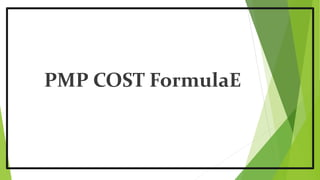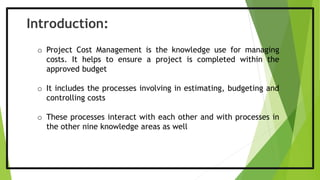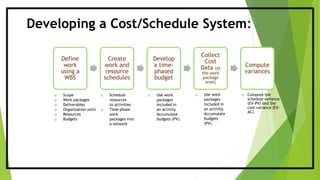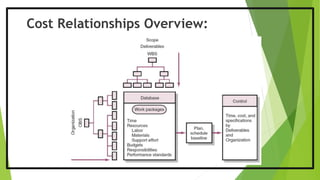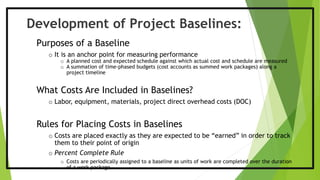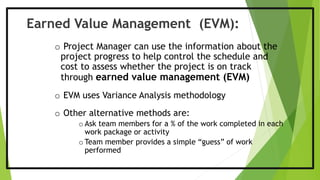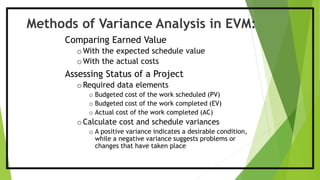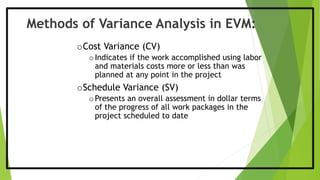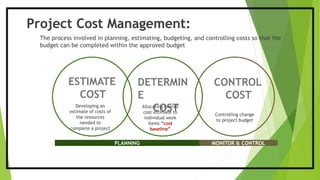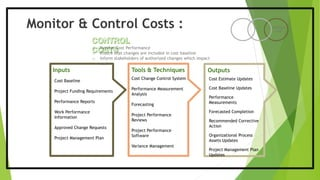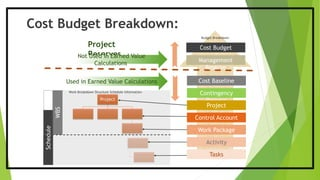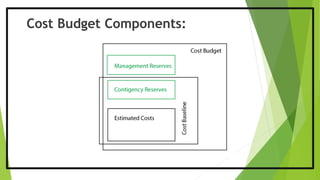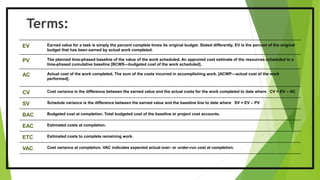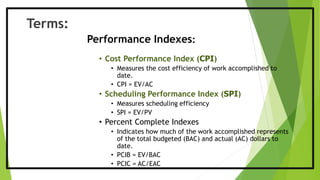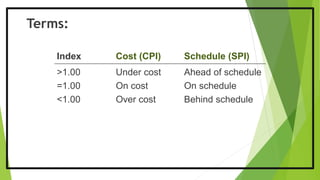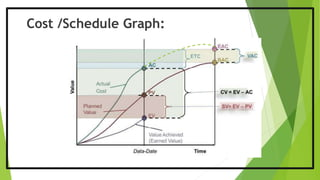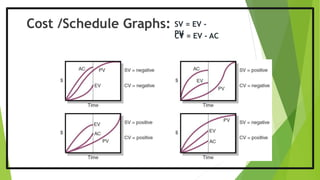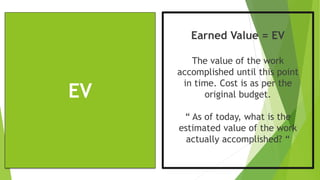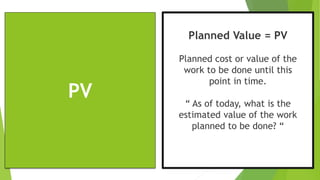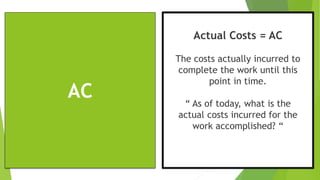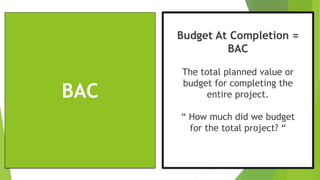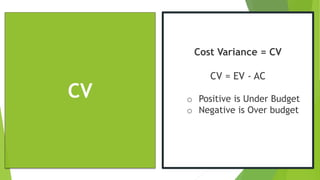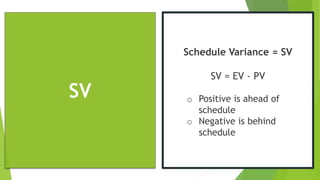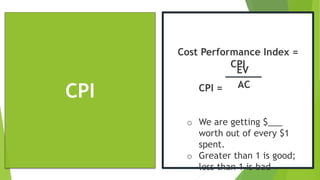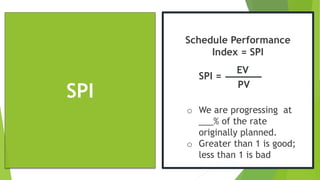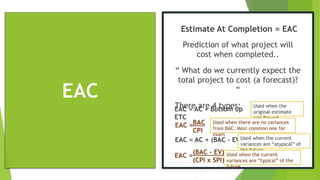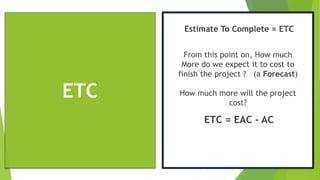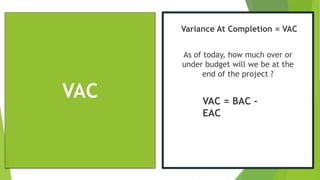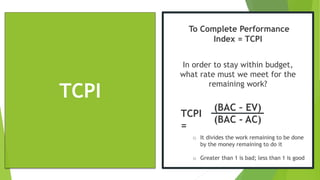1 von 30
Anzeige

### PMP COST FORMULAE

1. PMP COST FormulaE
2. Introduction: o Project Cost Management is the knowledge use for managing costs. It helps to ensure a project is completed within the approved budget o It includes the processes involving in estimating, budgeting and controlling costs o These processes interact with each other and with processes in the other nine knowledge areas as well
3. Developing a Cost/Schedule System: Define work using a WBS Create work and resource schedules Develop a time- phased budget Collect Cost Data (at the work package level) Compute variances o Scope o Work packages o Deliverables o Organization units o Resources o Budgets o Schedule resources to activities o Time-phase work packages into a network o Use work packages included in an activity. Accumulate budgets (PV). o Use work packages included in an activity. Accumulate budgets (PV) o Compute the schedule variance (EV-PV) and the cost variance (EV- AC)
4. Cost Relationships Overview:
5. Development of Project Baselines: Purposes of a Baseline o It is an anchor point for measuring performance o A planned cost and expected schedule against which actual cost and schedule are measured o A summation of time-phased budgets (cost accounts as summed work packages) along a project timeline What Costs Are Included in Baselines? o Labor, equipment, materials, project direct overhead costs (DOC) Rules for Placing Costs in Baselines o Costs are placed exactly as they are expected to be “earned” in order to track them to their point of origin o Percent Complete Rule o Costs are periodically assigned to a baseline as units of work are completed over the duration of a work package
6. o Project Manager can use the information about the project progress to help control the schedule and cost to assess whether the project is on track through earned value management (EVM) o EVM uses Variance Analysis methodology o Other alternative methods are: o Ask team members for a % of the work completed in each work package or activity o Team member provides a simple “guess” of work performed Earned Value Management (EVM):
7. Methods of Variance Analysis in EVM: Comparing Earned Value oWith the expected schedule value oWith the actual costs Assessing Status of a Project oRequired data elements o Budgeted cost of the work scheduled (PV) o Budgeted cost of the work completed (EV) o Actual cost of the work completed (AC) oCalculate cost and schedule variances o A positive variance indicates a desirable condition, while a negative variance suggests problems or changes that have taken place
8. oCost Variance (CV) oIndicates if the work accomplished using labor and materials costs more or less than was planned at any point in the project oSchedule Variance (SV) oPresents an overall assessment in dollar terms of the progress of all work packages in the project scheduled to date Methods of Variance Analysis in EVM:
9. Project Cost Management: ESTIMATE COST DETERMIN E COST CONTROL COST The process involved in planning, estimating, budgeting, and controlling costs so that the budget can be completed within the approved budget Developing an estimate of costs of the resources needed to complete a project Allocating overall cost estimate to individual work items “cost baseline” Controlling change to project budget PLANNING MONITOR & CONTROL
10. Monitor & Control Costs : CONTROL COST Cost Baseline Project Funding Requirements Performance Reports Work Performance Information Approved Change Requests Project Management Plan Cost Change Control System Performance Measurement Analysis Forecasting Project Performance Reviews Project Performance Software Variance Management Inputs Tools & Techniques Outputs Cost Estimate Updates Cost Baseline Updates Performance Measurements Forecasted Completion Recommended Corrective Action Organizational Process Assets Updates Project Management Plan Updates o Monitor Cost Performance o Ensure that changes are included in cost baseline o Inform stakeholders of authorized changes which impact cost
11. Cost Budget Breakdown: Project ReservesNot Used in Earned Value Calculations Used in Earned Value Calculations Cost Budget Management Reserve Cost Baseline Contingency Reserve Project Control Account Work Package Activity Tasks Project WBS Schedule Work Breakdown Structure Schedule Information Budget Breakdown
12. Cost Budget Components:
13. Terms: EV Earned value for a task is simply the percent complete times its original budget. Stated differently, EV is the percent of the original budget that has been earned by actual work completed. PV The planned time-phased baseline of the value of the work scheduled. An approved cost estimate of the resources scheduled in a time-phased cumulative baseline [BCWS—budgeted cost of the work scheduled]. AC Actual cost of the work completed. The sum of the costs incurred in accomplishing work. [ACWP—actual cost of the work performed]. CV Cost variance is the difference between the earned value and the actual costs for the work completed to date where CV = EV – AC SV Schedule variance is the difference between the earned value and the baseline line to date where SV = EV – PV BAC Budgeted cost at completion. Total budgeted cost of the baseline or project cost accounts. EAC Estimated costs at completion. ETC Estimated costs to complete remaining work. VAC Cost variance at completion. VAC indicates expected actual over- or under-run cost at completion.
14. Terms: Performance Indexes: • Cost Performance Index (CPI) • Measures the cost efficiency of work accomplished to date. • CPI = EV/AC • Scheduling Performance Index (SPI) • Measures scheduling efficiency • SPI = EV/PV • Percent Complete Indexes • Indicates how much of the work accomplished represents of the total budgeted (BAC) and actual (AC) dollars to date. • PCIB = EV/BAC • PCIC = AC/EAC
15. Terms: Index Cost (CPI) Schedule (SPI) >1.00 Under cost Ahead of schedule =1.00 On cost On schedule <1.00 Over cost Behind schedule
16. Cost /Schedule Graph:
17. Cost /Schedule Graphs: SV = EV - PVCV = EV - AC
18. EV Earned Value = EV The value of the work accomplished until this point in time. Cost is as per the original budget. “ As of today, what is the estimated value of the work actually accomplished? “
19. PV Planned Value = PV Planned cost or value of the work to be done until this point in time. “ As of today, what is the estimated value of the work planned to be done? “
20. AC Actual Costs = AC The costs actually incurred to complete the work until this point in time. “ As of today, what is the actual costs incurred for the work accomplished? “
21. BAC Budget At Completion = BAC The total planned value or budget for completing the entire project. “ How much did we budget for the total project? “
22. CV Cost Variance = CV CV = EV - AC o Positive is Under Budget o Negative is Over budget
23. SV Schedule Variance = SV SV = EV - PV o Positive is ahead of schedule o Negative is behind schedule
24. CPI Cost Performance Index = CPI CPI = o We are getting \$___ worth out of every \$1 spent. o Greater than 1 is good; less than 1 is bad EV AC
25. SPI Schedule Performance Index = SPI SPI = o We are progressing at ___% of the rate originally planned. o Greater than 1 is good; less than 1 is bad EV PV
26. EAC Estimate At Completion = EAC Prediction of what project will cost when completed.. “ What do we currently expect the total project to cost (a forecast)? “ There are 4 types:EAC = AC + Bottom up ETC BAC CPI EAC = AC + (BAC – EV) EAC = EAC = (BAC – EV) (CPI x SPI) Used when the original estimate was flawed Used when there are no variances from BAC. Most common one for exam Used when the current variances are “atypical” of the future Used when the current variances are “typical” of the future
27. ETC Estimate To Complete = ETC From this point on, How much More do we expect it to cost to finish the project ? (a Forecast) How much more will the project cost? ETC = EAC - AC
28. VAC Variance At Completion = VAC As of today, how much over or under budget will we be at the end of the project ? VAC = BAC - EAC
29. TCPI To Complete Performance Index = TCPI In order to stay within budget, what rate must we meet for the remaining work? TCPI = (BAC – EV) (BAC - AC) o It divides the work remaining to be done by the money remaining to do it o Greater than 1 is bad; less than 1 is good
30. Thank You Connect with me @ @
Anzeige